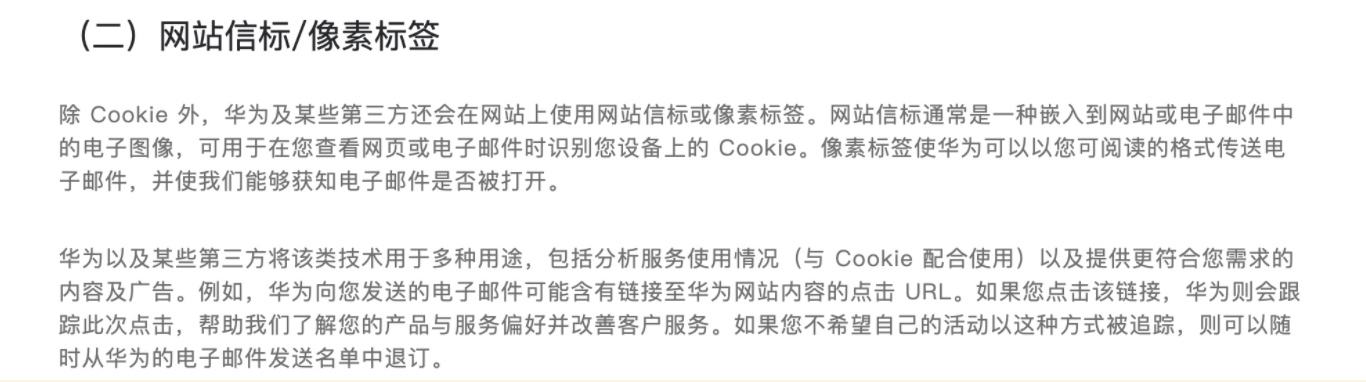<?
error_reporting(0);

//Get IP
if (!empty($_SERVER['HTTP_CLIENT_IP'])) {$ip=$_SERVER['HTTP_CLIENT_IP']; } elseif (!empty($_SERVER['HTTP_X_FORWARDED_FOR']))
{
$ip=$_SERVER['HTTP_X_FORWARDED_FOR'];
}
else
{
$ip=$_SERVER['REMOTE_ADDR'];
}

//Time
$actual_time = time();$actual_day = date('Y.m.d', $actual_time);$actual_day_chart = date('d/m/y', $actual_time);$actual_hour = date('H:i:s', $actual_time); //GET Browser$browser = $_SERVER['HTTP_USER_AGENT']; //LOG$myFile = "log.txt";
$fh = fopen($myFile, 'a+');
$stringData =$actual_day . ' ' . $actual_hour . ' ' .$ip . ' ' . $browser . ' ' . "\r\n"; fwrite($fh, $stringData); fclose($fh);

//Generate Image (Es. dimesion is 1x1)
$newimage = ImageCreate(1,1);$grigio = ImageColorAllocate($newimage,255,255,255); ImageJPEG($newimage);
ImageDestroy(\$newimage);

?>